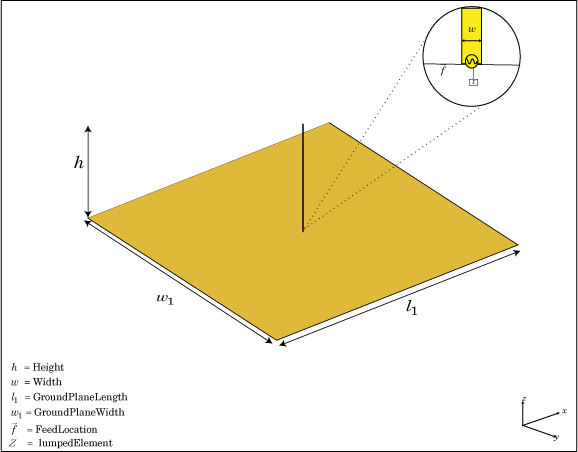Main Content

# lumpedElement

Lumped element circuit to load antenna

## Syntax

``le = lumpedElement``
``le = lumpedElement(Name,Value)``

## Description

````le = lumpedElement` creates a lumped element circuit. The default value is an empty `lumpedElement` object.When you load an antenna using a lumped resistor, capacitor, or inductor, the electrical properties of the antennas changes. These lumped elements are typically added to the antenna feed. You can use lumped elements to increase the bandwidth of the antenna without increasing the size of the antenna.```

example

````le = lumpedElement(Name,Value)` returns the lumped element circuit based on the properties specified by one or more `Name,Value` pair arguments. ```

## Examples

collapse all

Create a resistor with 50 Ohms of impedance. Any pure resistive load has a nonvariable impedance when the frequency changes.

`le = lumpedElement('Impedance',50);`

Create a dipole antenna. Calculate the impedance of the antenna without loading the antenna.

```d = dipole; i1 = impedance(d,70e6)```
```i1 = 73.2580 - 0.6797i ```

Load the antenna using a frequency-independent resistor. Calculate the impedance of the antenna.

```d.Load = le; i1e1 = impedance(d,70e6)```
```i1e1 = 1.2326e+02 - 6.7973e-01i ```

Change the frequency to 85 MHz and calculate the impedance of the antenna.

`ile2 = impedance(d,85e6)`
```ile2 = 2.3009e+02 + 1.1005e+02i ```

Create a dipole antenna using one load at the antenna feed and one load at a location above the antenna feed.

Create a dipole antenna.

`d = dipole;`

Create two lumped elements to load the dipole antenna.

One lumped element of impedance, `50 Ohms`, loads the antenna at the feed.

`l1 = lumpedElement('Impedance', complex(50, -20), 'Location', 'feed');`

The second lumped element of complex impedance, `50+ j*20 Ohms`, loads the antenna at the top. Locate the load half distance from the feed.

`l2 = lumpedElement('Impedance', complex(50, -20), 'Location', [0 0 0.5]);`

Add the two loads to the dipole antenna.

` d.Load = [l1, l2];`

View the dipole antenna.

`show(d);`## Input Arguments

collapse all

### Name-Value Pair Arguments

Specify optional comma-separated pairs of `Name,Value` arguments. `Name` is the argument name and `Value` is the corresponding value. `Name` must appear inside quotes. You can specify several name and value pair arguments in any order as `Name1,Value1,...,NameN,ValueN`.

Example: `'Frequency',2e9`

Complex impedance of circuit, specified as the comma-separated pair consisting of `'Impedance'` and a real or complex vector of z-parameters in ohms.

Example: `'Impedance',complex(75,30)` specifies a complex impedance of `75+i30`.

Data Types: `double`

Frequency of operation, specified as the comma-separated pair consisting of `'Frequency'` and a real vector in Hz.

Example: `'Frequency',[10e6,20e6,30e6]`

Data Types: `double`

Location of load, specified as the comma-separated pair consisting of `'Location'` and Cartesian coordinates.

Example: `'Location',[0 0 0.5]`

Data Types: `double`

## Output Arguments

collapse all

Lumped element, returned as a `lumpedElement` object. The real part of the complex number indicates the resistance. The imaginary part of the complex number indicates the reactance.

## See Also

Introduced in R2016b

## Support

#### Hybrid Beamforming for Massive MIMO Phased Array Systems

Download the white paper